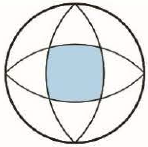Chapter 10, Problem 1P

Chapter
Section
Textbook Problem

# The outer circle in the figure has radius 1 and the centers of the interior circular arcs lie on the outer circle. Find the area of the shaded region.FIGURE FOR PROBLEM 1To determine

To find: The area of the shaded region from the given circle.

Explanation

Given:

The radius of the outer circle is 1.

Calculation:

Draw the figure with radius as shown in figure (1).

Calculate the value of radius r using the formula.

r=12+12r=2

Write the equation for the shaded region.

x2+y2=(2)2

(x+1)2+(y+0)2=2(x+1)2+y2=2

Substitute rcosθ for x and rsinθ for y in the above equation.

(x+1)2+y2=2(rcosθ+1)2+(rsinθ)2=2[r2cos2θ+1+2rcosθ]+r2sin2θ=2r2(cos2θ+sin2θ)+1+2rcosθ=2r2(1)+1+2rcosθ=2r2+1+2rcosθ=2r2+2rcosθ=21r2+2rcosθ1=0or,r2=12rcosθ

Calculate the value of r from the above equation using quadratic formula.

r=2cosθ±(2cosθ)2+4(1)(1)2(1)=2cosθ±4cos2θ+4(1)(1)2(1)=(2cosθ+2cos2+1)2=2(cosθ+cos2+1)2r=cosθ+cos2+1

Here, from the above figure, the shaded region is 18th of the entire shaded area.

Calculate the area of the shaded region.

Here, the considered shaded region contains limits of 0 to 45 degree that is 0π4

18A=120π4r2dθ

Substitute 12rcosθ for r2 in the above equation.

18A=120π4r2dθ14A=0π4(12rcosθ)dθ

Substitute cosθ+cos2+1 for r in the above equation.

14A=0π4{12cosθ(cosθ+cos2+1)}dθ

Substitute 12(cos2θ+1) for cos2θ in the above equation

### Still sussing out bartleby?

Check out a sample textbook solution.

See a sample solution

#### The Solution to Your Study Problems

Bartleby provides explanations to thousands of textbook problems written by our experts, many with advanced degrees!

Get Started

#### Evaluate the limit, if it exists. limh0(x+h)x3h

Single Variable Calculus: Early Transcendentals, Volume I

#### In Problems 45-51, solve each system of equations. 51.

Mathematical Applications for the Management, Life, and Social Sciences

#### True or False: converges if and only if .

Study Guide for Stewart's Multivariable Calculus, 8th

#### The graph at the right has a local maximum at x ______. a) 1, 3, and 5 b) 2 c) 4 d) 2 and 4

Study Guide for Stewart's Single Variable Calculus: Early Transcendentals, 8th